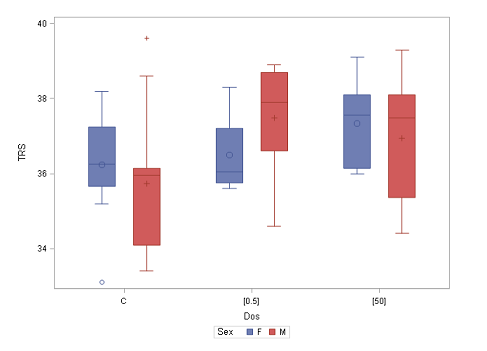25
Apr

–

11
May
Alnarp

# Statistics I: Basic statistics, 4 hpThe Ph. D. course Statistics I - Basic Statistics, 4 hp, will be given in Alnarp in April-May 2022.

### Objective, including learning outcomes

The objective of the course is to give an overview of the basic ideas and terminology in statistics and to learn how to use the computer to do a statistical analysis.

On completion of the course, the student will be able to:

• make graphical and numerical descriptions of data
• apply basic probability concepts and statistical principles
• design and formulate an experiment in statistical terms
• describe common statistical methods including conditions and assumptions
• using the computer to carry out a statistical analysis in scientific research
• interpret and evaluate results correctly and draw reasonable conclusions
• clearly and concisely communicate results and conclusions

### Content

The course is built on lectures and computer exercises. The main moments are as follows:

• Terminology in probability and statistics.
• Descriptive statistics.
• Basic probability theory.
• Research design.
• Statistical inference of means and proportions.
• Basic ideas of regression and correlation.
• Basic ideas of nonparametric methods.

## Facts

Time: 2022-04-25 - 2022-05-11
City: Alnarp
Last signup date: 11 April 2022